Refer to our Texas Go Math Grade 2 Answer Key Pdf to score good marks in the exams. Test yourself by practicing the problems from Texas Go Math Grade 2 Lesson 10.2 Answer Key 3-Digit Addition: Regroup Ones.

Explore

Use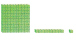to model the problem. Draw quick pictures to show what you did.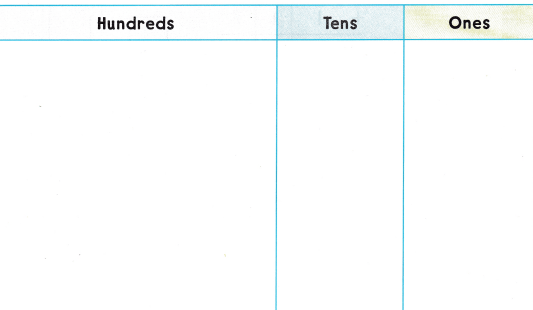FOR THE TEACHER • Read the following problem and have children model it with blocks, There were 213 people at the show on Friday and 156 people at the show on Saturday. How many people were at the show on the two nights? Have children draw quick pictures to show how they solved the problem.Math Talk
Mathematical Processes

Describe how you modeled the problem.
I drew 3 hundred blocks, 6 ten blocks and 9 one blocks.

Model and Show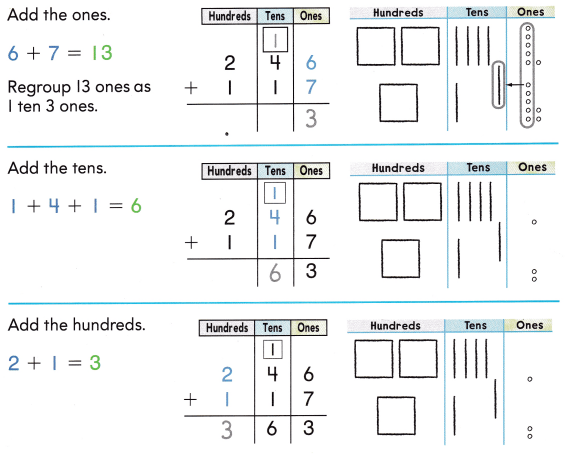Share and Show

Write the sum.

Question 1.Explanation:
The sum of 328 and 134 is 462.

Question 2.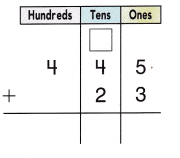Explanation:
The sum of 445 and 23 is 468.

Problem Solving

Write the sum.

Question 3.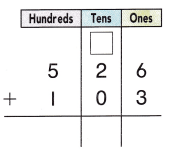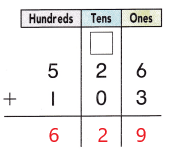Explanation:
The sum of 526 and 103 is 629.

Question 4.Explanation:
The sum of  348 and 19 is 367.

Question 5.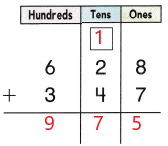Explanation:
The sum of 628 and 347 is 975.

Question 6.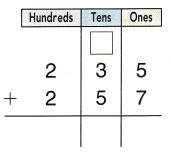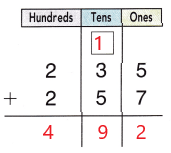Explanation:
The sum of 235 and 257 is 492.

Solve. Write or draw to explain.

Question 7.
Multi-Step On Thursday, there were 326 visitors at the zoo. There were 200 more visitors at the zoo on Friday than on Thursday. How many visitors were at the zoo on both days?__________ visitors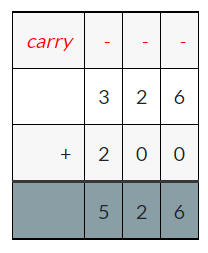Explanation:
The sum of 326 and 200 is 526
So, 526 visitors are at the Zoo on both days.

Question 8.
H.O.T. The zoo train stop is 235 steps away from the gift shop. Julia walks to the gift shop and back. How many total steps is this?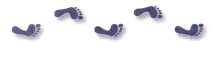_____________ steps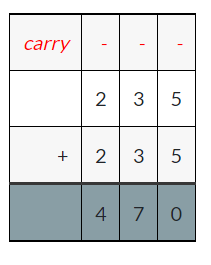Explanation:
The sum of 235 and 235 is 470
So, the total number of steps are 470.

Question 9.
On Monday, 328 penguins leave to travel north. On Tuesday, 433 penguins leave. How many penguins leave in these two days?
(A) 760
(B) 761
(C) 751
(B) 761

Explanation:
On Monday, 328 penguins leave to travel north
On Tuesday, 433 penguins leave
328 + 433 = 761
So, 761 penguins left in these two days.

Question 10.
Communicate One day 575 seals swim away. The next day. 415 seals swim away. How many seals swim away in the two days?
(A) 990
(B) 995
(C) 980
(A) 990

Explanation:
Communicate One day 575 seals swim away
The next day  415 seals swim away
575 + 415 = 990
So, 990 seals swam away in the two days.

Question 11.
Reasoning Fish travel in large groups. One group has 35 fish. Another group has 524 fish. How many fish are in the two groups?
(A) 883
(B) 873
(C) 893

Question 12.
TEXAS Test Prep On Thursday, 175 drinks were sold at the zoo. On Friday. 219 drinks were sold. How many drinks were sold on both days?
(A) 484
(B) 394
(C) 404
(B) 394

Explanation:
On Thursday, 175 drinks were sold at the zoo
On Friday 219 drinks were sold
175 + 219 = 394
So, 394 drinks were sold on both days.

TAKE HOME ACTIVITY • Ask your child to explain why he or she. regrouped in only some of the problems in this lesson.

### Texas Go Math Grade 2 Lesson 10.2 Homework and Practice Answer Key

Write the sum.

Question 1.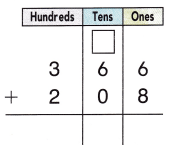Explanation:
The sum of 366 and 208 is 574.

Question 2.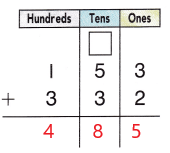Explanation:
The sum of 153 and 332 is 485.

Question 3.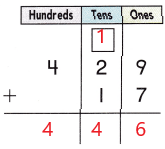Explanation:
The sum of 429 and 17 is 446.

Question 4.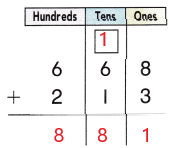Explanation:
The sum of 668 and 213 is 881.

Problem Solving

Choose a way to solve. Write or draw to explain.

Question 5.
Multi-Step On Monday, there were 114- children at the park. There were 150 more children at the park on Tuesday than on Monday. How many children were at the park on both days?
_________ children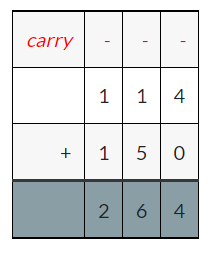Explanation:
The sum of 114 and 150 is 264
So, 264 children were at the park on both days.

Lesson Check

Question 6.
On Saturday, 155 pumpkins were sold at the town fair. On Sunday, 138 pumpkins were sold. How many pumpkins were sold on both days?
(A) 293
(B) 285
(C) 283
(A) 293

Explanation:
On Saturday, 155 pumpkins were sold at the town fair
On Sunday 138 pumpkins were sold
155 + 138 = 293
So, 293 pumpkins were sold on both days.

Question 7.
There were 229 fish in a large fish tank. Another large fish tank has 214 fish. How many fish are there in the two tanks?
(A) 443
(B) 433
(C) 444
(A) 443

Explanation:
There were 229 fish in a large fish tank
Another large fish tank has 214 fish
229 + 214 = 443
So, 443 fishes are there in the two tanks.

Question 8.
On Thursday, 937 workers travel by train to work. On Friday, 353 workers travel by train to work. How many workers traveled by train on these two days?
(A) 780
(B) 790
(C) 784

Question 9.
The bus stop is 227 steps away from Karen’s house. One morning she walks to the bus stop. After school, she walks from the bus stop to her house. How many steps does she walk to and from the bus stop?
(A) 444
(B) 457
(C) 454